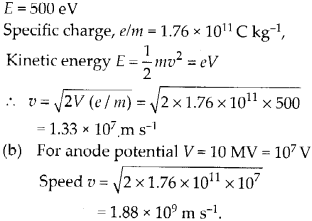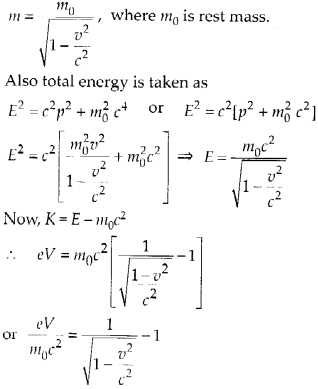Enlightened

# Question 20: NCERT Solutions for 12th Class Physics: Chapter 11-Dual Nature of Radiation and Matter

• 0

Question 20: NCERT Solutions for 12th Class Physics: Chapter 11-Dual Nature of Radiation and Matter

(a) Estimate the speed with which electrons emitted from a heated cathode of an evacuated tube impinge on the anode maintained at a potential difference of 500 V with respect to the cathode. Ignore the small initial speeds of the electrons. The ‘specific charge’ of the electron i.e., its e/m is given to be 1.76 × 1011 C kg-1.
(b) Use the same formula you employ in (a) to obtain electron speed for an anode potential of 10 MV. Do you see what is wrong? In what way is the formula to be modified?

Share

1. Solution:
(a) Energy of accelerated electronThis speed of electron is impossible. Since nothing can move with a speed greater than speed of light (c = 3 × 1o8 m s-1 The formula for kinetic energy E = 1/2 mv2 valid only for v « c. For the situation when speed v is comparable to speed of light c, we use relativistic formula. The relativistic mass is given bySubstituting the valuesCheck the complete chapter with solutions.
NCERT Solutions for 12th Class Physics: Chapter 11-Dual Nature of Radiation and Matter

• 0